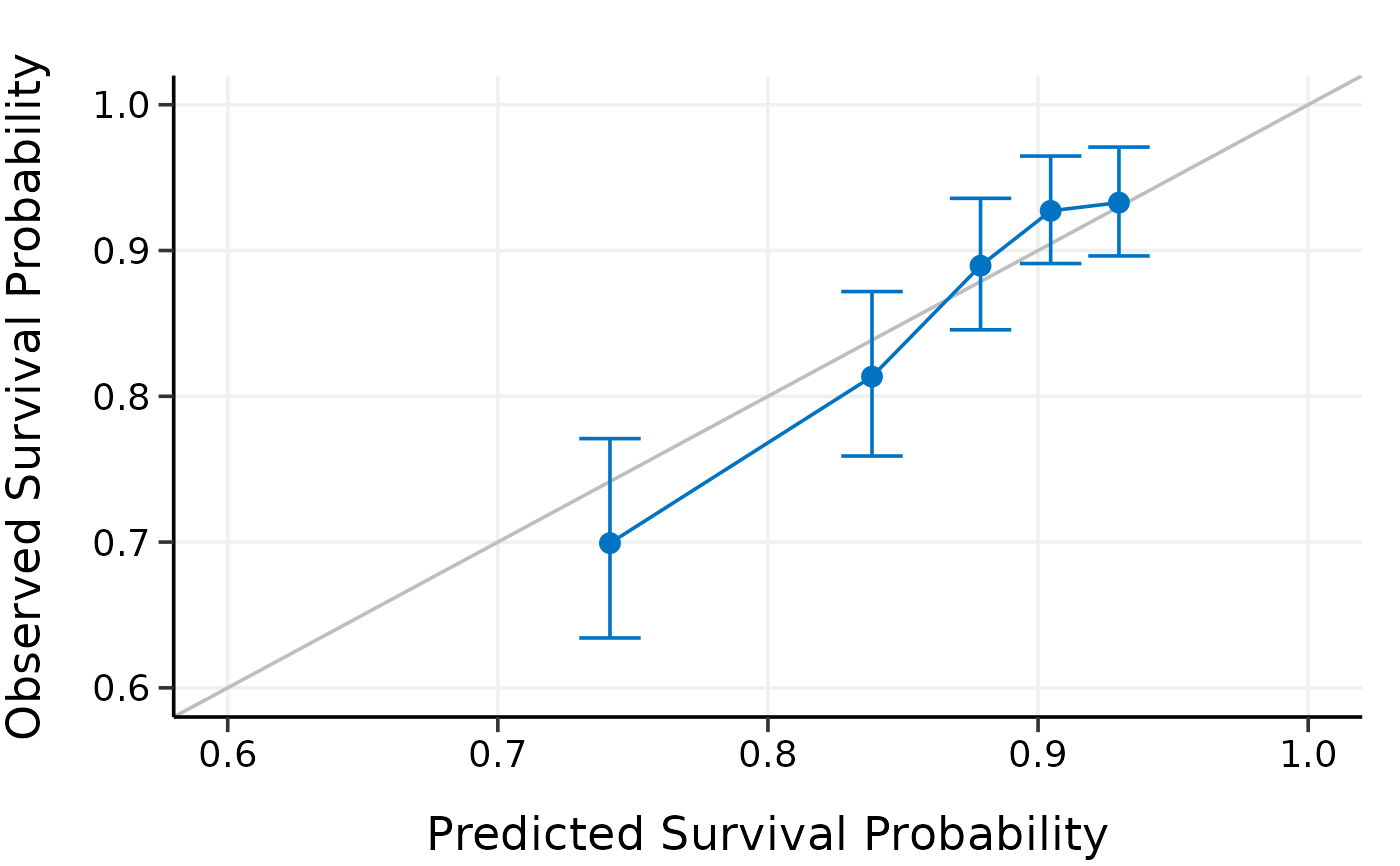Externally calibrate high-dimensional Cox models

calibrate_external(object, x, time, event, x_new, time_new, event_new,
pred.at, ngroup = 5)

## Arguments

object Model object fitted by hdnom::fit_*(). Matrix of training data used for fitting the model. Survival time of the training data. Must be of the same length with the number of rows as x. Status indicator of the training data, normally 0 = alive, 1 = dead. Must be of the same length with the number of rows as x. Matrix of predictors for the external validation data. Survival time of the external validation data. Must be of the same length with the number of rows as x_new. Status indicator of the external validation data, normally 0 = alive, 1 = dead. Must be of the same length with the number of rows as x_new. Time point at which external calibration should take place. Number of groups to be formed for external calibration.

## Examples

library("survival")

# Load imputed SMART data
data(smart)
# Use the first 1000 samples as training data
# (the data used for internal validation)
x <- as.matrix(smart[, -c(1, 2)])[1:1000, ]
time <- smart$TEVENT[1:1000] event <- smart$EVENT[1:1000]

# Take the next 1000 samples as external calibration data
# In practice, usually use data collected in other studies
x_new <- as.matrix(smart[, -c(1, 2)])[1001:2000, ]
time_new <- smart$TEVENT[1001:2000] event_new <- smart$EVENT[1001:2000]

# Fit Cox model with lasso penalty
fit <- fit_lasso(
x, Surv(time, event),
nfolds = 5, rule = "lambda.1se", seed = 11
)

# External calibration
cal.ext <- calibrate_external(
fit, x, time, event,
x_new, time_new, event_new,
pred.at = 365 * 5, ngroup = 5
)

print(cal.ext)#> High-Dimensional Cox Model External Calibration Object
#> Model type: lasso
#> Calibration time point: 1825
#> Number of groups formed for calibration: 5 summary(cal.ext)#>   External Calibration Summary Table
#>   Predicted  Observed Lower 95% Upper 95%
#> 1 0.7867982 0.6822816 0.6167482 0.7547785
#> 2 0.8289112 0.8308652 0.7775687 0.8878148
#> 3 0.8542307 0.9122714 0.8741931 0.9520084
#> 4 0.8751486 0.9005033 0.8589700 0.9440448
#> 5 0.8949158 0.9452770 0.9103990 0.9814912plot(cal.ext, xlim = c(0.6, 1), ylim = c(0.6, 1))# # Test fused lasso, MCP, and Snet models
# data(smart)
# # Use first 500 samples as training data
# # (the data used for internal validation)
# x <- as.matrix(smart[, -c(1, 2)])[1:500, ]
# time <- smart$TEVENT[1:500] # event <- smart$EVENT[1:500]
#
# # Take 1000 samples as external validation data.
# # In practice, usually use data collected in other studies.
# x_new <- as.matrix(smart[, -c(1, 2)])[1001:2000, ]
# time_new <- smart$TEVENT[1001:2000] # event_new <- smart$EVENT[1001:2000]
#
# flassofit <- fit_flasso(x, survival::Surv(time, event), nfolds = 5, seed = 11)
# scadfit <- fit_mcp(x, survival::Surv(time, event), nfolds = 5, seed = 11)
# mnetfit <- fit_snet(x, survival::Surv(time, event), nfolds = 5, seed = 11)
#
# cal.ext1 <- calibrate_external(
#   flassofit, x, time, event,
#   x_new, time_new, event_new,
#   pred.at = 365 * 5, ngroup = 5)
#
# cal.ext2 <- calibrate_external(
#   scadfit, x, time, event,
#   x_new, time_new, event_new,
#   pred.at = 365 * 5, ngroup = 5)
#
# cal.ext3 <- calibrate_external(
#   mnetfit, x, time, event,
#   x_new, time_new, event_new,
#   pred.at = 365 * 5, ngroup = 5)
#
# print(cal.ext1)
# summary(cal.ext1)
# plot(cal.ext1)
#
# print(cal.ext2)
# summary(cal.ext2)
# plot(cal.ext2)
#
# print(cal.ext3)
# summary(cal.ext3)
# plot(cal.ext3)